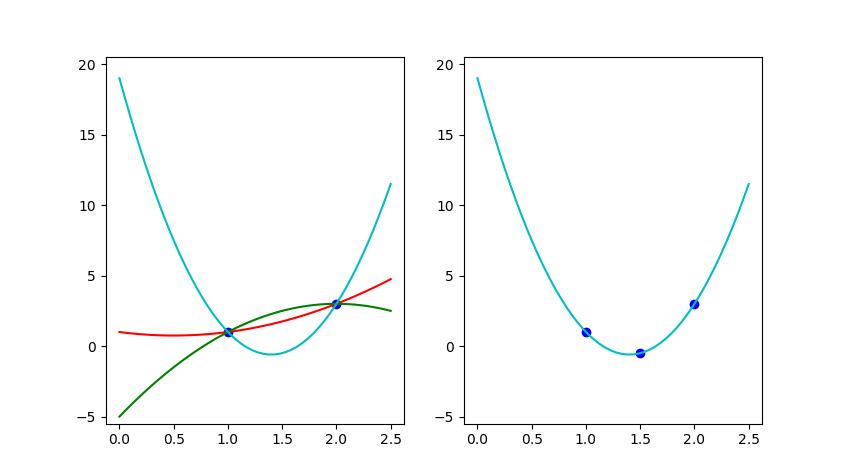# Thresholdizing Lattice-Based Encryption Schemes

## Context / Abstract

In this thesis, we apply secret sharing techniques on lattice based encryption schemes, namely fully homomorphic encryption and predicate encryption. Using secret sharing techniques from [BGG+17], we show how to construct paradigms of threshold multi-key fully homomorphic encryption and predicate encryption. Through multi-key fully homomorphic encryption and threshold fully homomorphic encryption, we can construct a low-round multi party computation (MPC) scheme with guaranteed output delivery, assuming honest majority in the semi-honest and malicious settings. Applying the secret sharing scheme on predicate encryption constructions from LWE, we can obtain a distributed predicate encryption scheme.

My thesis is available here.

I would like to extend special thanks to my advisor Yael Kalai my collaborator Adam Sealfon.

Below, I’d like to give a high level overview of some of the cryptographic concepts. For more technical details, please see my thesis or the links to the courses below!

## Secret Sharing

Secret sharing is a cryptographic protocol that involves sharing a secret among a group of participants (each receiving shares of the secret), such that the secret can only be reconstructed if a sufficient number of parties combine their shares. The motivation of this primitive is from cryptographic key management, in which storing a secret key would be more secure if split among multiple parties.

More formally, a secret sharing scheme has two algorithms, $Share$ and $Reconstruct$:

• $Share(s, \mathbb{A}) \to (s_1, \dots s_N)$: The share algorithm takes in input secret $s$, an access structure $\mathbb{A}$ for $N$ parties, and outputs $N$ shares in which share $s_i$ is sent to party $i$.
• $Reconstruct(S) \to s$: The reconstruct algorithm takes in a set of shares $S$ such that if number of shares in $S$ is sufficient relative to the access structure, then the secret $s$ is returned. Otherwise, $\perp$ is returned.

The properties that we want out of a secret sharing scheme are:

• Correctness: if a sufficient number of shares are given to the reconstruction algorithm, then the secret will be recovered.
• Security: if an insufficient number of shares are given to the reconstruction algorithm, then the secret should not be recovered.

One of the most famous examples of secret sharing is Shamir’s Secret Sharing Scheme which uses the elegant idea of polynomial interpolation to achieve correctness and privacy. A secret $s$ is simply the $y$ intercept of a degree $n-1$ polynomial $f(x)$, and party $i$ is given $f(i)$ as her share. If $n$ parties combine their shares, through polynomial interpolation it is possible to uniquely reconstruct $s$. However, if there are fewer than $n$ shares available, then it is impossible to uniquely reconstruct $f(x)$ and determine the secret!Left: unable to determine the polynomial with only two points. Right: uniquely determining a degree 2 polynomial with three points. Source of image.

## Fully Homomorphic Encryption

Fully Homomorphic Encryption is a recently realized construction which I think is really cool! In essence, it allows computation on encrypted data the same way we would compute on plaintext. An encryption scheme is a family algorithms consisting of $KeyGen$ (which generates the public and secret key, $Enc$ (which takes in as input the public key and a plaintext message and outputs a ciphertext) and $Dec$ (which uses the secret key to decrypt a ciphertext and output a message).

In FHE, we allow an additional $Eval$ algorithm. Assume that $ct_1 = Enc(m_1)$ and $ct_2 = Enc(m_2)$. We can generate $ct^+ = Add(ct_1, ct_2)$ such that $Dec(ct^+) = m_1 + m_2$. We can also generate $ct^* = Mul(ct_1, ct_2)$ such that $Dec(ct^+) = m_1 * m_2$. With addition and multiplication, we can simulate any circuit and this any function! In theory, if you gave your data encrypted to Google, Google could use FHE to run their algorithms on your data, and return to you the queries you desire, without them learning anything about your underlying data!

## Predicate Encryption

Predicate Encryption is another type of augmentation of classical encryption schemes. A ciphertext $ct$ is now associated with a private set of attributes $x$ such that one holding a secret key $sk_C$ associated with predicate $C$ can only decrypt $ct$ if $C(x) = 1$. Predicate encryption is a generalization of attribute-based encryption but is also a special case of functional encryption.

Predicate encryption has many interesting applications. For example, one may wish to encrypt the location of a club meeting with the attributes such that only people who can prove their identity may decrypt and discover the location.

## My Thesis, in summary

Using secret sharing techniques, we can secret share the secret key of predicate encrytion and fully homomorphic encryption schemes to allow for decentralized decryption. This means that, we can have $k$ parties output partial decryptions of a FHE or PE scheme and be able to reconstruct the output using the partial decryptions, while preserving the secrecy of the secret key shares. Applications of these techniques include low round multi-party computation and decentralized predicate encryption.